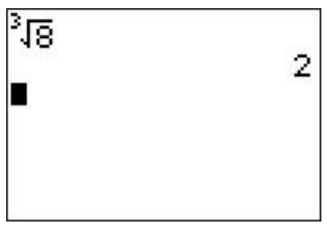# 26.7: A.7- Special functions (absolute value, n-th root, etc.)

$$\newcommand{\vecs}{\overset { \rightharpoonup} {\mathbf{#1}} }$$ $$\newcommand{\vecd}{\overset{-\!-\!\rightharpoonup}{\vphantom{a}\smash {#1}}}$$$$\newcommand{\id}{\mathrm{id}}$$ $$\newcommand{\Span}{\mathrm{span}}$$ $$\newcommand{\kernel}{\mathrm{null}\,}$$ $$\newcommand{\range}{\mathrm{range}\,}$$ $$\newcommand{\RealPart}{\mathrm{Re}}$$ $$\newcommand{\ImaginaryPart}{\mathrm{Im}}$$ $$\newcommand{\Argument}{\mathrm{Arg}}$$ $$\newcommand{\norm}{\| #1 \|}$$ $$\newcommand{\inner}{\langle #1, #2 \rangle}$$ $$\newcommand{\Span}{\mathrm{span}}$$ $$\newcommand{\id}{\mathrm{id}}$$ $$\newcommand{\Span}{\mathrm{span}}$$ $$\newcommand{\kernel}{\mathrm{null}\,}$$ $$\newcommand{\range}{\mathrm{range}\,}$$ $$\newcommand{\RealPart}{\mathrm{Re}}$$ $$\newcommand{\ImaginaryPart}{\mathrm{Im}}$$ $$\newcommand{\Argument}{\mathrm{Arg}}$$ $$\newcommand{\norm}{\| #1 \|}$$ $$\newcommand{\inner}{\langle #1, #2 \rangle}$$ $$\newcommand{\Span}{\mathrm{span}}$$$$\newcommand{\AA}{\unicode[.8,0]{x212B}}$$

We now point out some important functions in the MATH menu ($$\boxed{\text{math}}$$) and the LIST menu ($$\boxed{\text{2nd}}$$$$\boxed{\text{stat}}$$), that are used in this course.

## Fractions

Suppose in the main screen you evaluate $$4/6$$. Your calculator will display $$.66666666667$$. If you wanted to see this as a fraction you would type $$\boxed{\text{math}}$$$$\boxed{\text{enter}}$$$$\boxed{\text{enter}}$$. You will now see $$2/3$$ displayed.

## Absolute value

To evaluate $$|-4|$$ you would type in the main screen $$\boxed{\text{math}}$$$$\boxed{\triangleright}$$$$\boxed{\text{enter}}$$$$\boxed{\text{(-)}}$$$$\boxed{\text{4}}$$$$\boxed{\text{enter}}$$. The answer $$4$$ should be displayed. To graph $$|x-2|$$, go to the Y= screen ($$\boxed{y=}$$) and enter in the Y1 (for example) space $$\boxed{\text{math}}$$$$\boxed{\triangleright}$$$$\boxed{\text{enter}}$$$$\boxed{\text{X,T,}\theta,n}$$$$\boxed{\text{-}}$$$$\boxed{\text{2}}$$$$\boxed{\triangleright}$$$$\boxed{\text{enter}}$$.Now you can go to the graphing screen to see the graph.

## $$n$$-th root

To calculate the cube root of $$8$$. Press on the main screen $$\boxed{\text{3}}$$$$\boxed{\text{math}}$$$$\boxed{\text{5}}$$ (or select the x-root) $$\boxed{\text{8}}$$$$\boxed{\text{enter}}$$.You can compute any root this way (e.g. the $$5$$th root of $$7$$). For the cube root you can also use $$\boxed{\text{math}}$$$$\boxed{\text{4}}$$ instead. Recall also the algebraic definition of the $$n$$th root,

$\sqrt[n]{x}=x^{\frac{1}{n}} \nonumber$

so that the $$5$$th root of $$7$$ can also be computed by pressing $$\boxed{\text{7}}$$$$\boxed{\wedge}$$$$\boxed{\text{(}}$$$$\boxed{\text{1}}$$$$\boxed{\div}$$$$\boxed{\text{5}}$$$$\boxed{\text{)}}$$.

## Factorials

To compute $$5!$$, for example, in the main screen type $$\boxed{\text{5}}$$$$\boxed{\text{math}}$$ then move the cursor to the right three times (or left once) so that the ’PRB’ menu is displayed. Option 4 is ’$$!$$’ so press $$\boxed{\text{4}}$$ or move the cursor to highlight this option and press $$\boxed{\text{enter}}$$. Finally press $$\boxed{\text{enter}}$$ and the answer $$120$$ will be displayed.## Combinations and permutations

To calculate $$_{5} C_{2}=\dbinom{5}{2}$$, in the main menu type $$\boxed{\text{5}}$$. Then go to the math-probability menu by pressing $$\boxed{\text{math}}$$ then the right (or left) arrow until ’PRB’ is highlighted. You will see that the third option is $$_nC_r$$ so press $$\boxed{\text{3}}$$. This will return you to the main screen. Press $$\boxed{\text{2}}$$$$\boxed{\text{enter}}$$. The answer $$10$$ will be displayed.Permutations $$_nP_r$$ are handled the same way except it is the second option under the math-probability menu instead of the third.

## Sequences and series

A sequence $$a_1,a_2,a_3,\dots$$ that is given by a formula can be added via the ‘LIST’ menu (press $$\boxed{\text{2nd}}$$$$\boxed{\text{stat}}$$). For example, to enter the first $$10$$ terms $$a_1,a_2,\dots, a_{10}$$ of the sequence $$a_n=n^2+1$$, we have to write $$seq(x^2+1,x,1,10)$$. Here, the ‘sequence’ command takes four inputs, first the assignment $$x^2+1$$, second the independent variable $$x$$, third the starting index $$1$$, and fourth the final index $$10$$. This expression is entered to the calculator by pressing $$\boxed{\text{2nd}}$$$$\boxed{\text{stat}}$$$$\boxed{\triangleright}$$$$\boxed{\text{5}}$$$$\boxed{\text{X,T,}\theta,n}$$$$\boxed{x^2}$$$$\boxed{\text{+}}$$$$\boxed{\text{1}}$$$$\boxed{\text{,}}$$$$\boxed{\text{X,T,}\theta,n}$$$$\boxed{\text{,}}$$$$\boxed{\text{1}}$$$$\boxed{\text{,}}$$$$\boxed{\text{1}}$$$$\boxed{\text{0}}$$$$\boxed{\text{)}}$$ and then confirmed by pressing $$\boxed{\text{enter}}$$. We obtain:To add the ten numbers $$a_1+\dots +a_{10}$$ of the sequence, we use the ‘sum’ command in the LIST-MATH menu. Press $$\boxed{\text{2nd}}$$$$\boxed{\text{stat}}$$$$\boxed{\triangleleft}$$$$\boxed{\text{5}}$$ to enter the ‘$$sum($$’ expression. Then using the previously entered answer by pressing $$\boxed{\text{2nd}}$$$$\boxed{\text{(-)}}$$, and finishing with $$\boxed{\text{)}}$$$$\boxed{\text{enter}}$$, we now calculate the wanted sum.This page titled 26.7: A.7- Special functions (absolute value, n-th root, etc.) is shared under a CC BY-NC-SA 4.0 license and was authored, remixed, and/or curated by Thomas Tradler and Holly Carley (New York City College of Technology at CUNY Academic Works) via source content that was edited to the style and standards of the LibreTexts platform; a detailed edit history is available upon request.